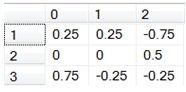# SQL Server matrix inverse

MATINVERSE

Updated: 31 January 2012

Use the scalar function MATINVERSE to calculate the matrix inverse of a square (N x N) array.
MATINVERSE expects a string representation of the matrix, with columns separated by commas and rows separated by semi-colons.
Syntax
SELECT [wctMath].[wct].[MATINVERSE](
<@Matrix1, nvarchar(max),>)
Arguments
@Matrix1
a string representation of a matrix.
Return Types
[nvarchar](max)
Remarks
·         The number of columns in the array must equal the number of rows in the array.
·         If the matrix determinant is equal to zero an error will be returned.
·         The string representations of the @Matrix1 must only contain numbers, commas to separate the columns, and semi-colons to separate the rows.
·         Consecutive commas will generate an error.
·         Consecutive semi-colons will generate an error.
·         Non-numeric data between commas will generate an error
·         Non-number data between semi-colons will generate an error
·         To convert non-normalized data to a string format, use the Matrix2String or the Matrix2String_q function.
·         To convert normalized data to a string format, us the NMatrix2String or the NMatrix2String_q function.
·         To convert the string result to a table, us the table-valued function MATRIX.
·         To calculate the matrix inverse directly on normalized data, use the table-valued functions MINVERSEN or MINVERSEN_q
·         To calculate matrix inverse directly on non-normalized data, us the table-valued functions MINVERSE or MINVERSE_q.
Examples
Let’s assume that we had the following matrix, A, and we want to calculate the inverse.
A = [1,2,1; 3,4,-1; 0,2,0]
We could enter the following SQL to perform the calculation.
DECLARE @A as varchar(max)

SET @A = '1,2,1; 3,4,-1; 0,2,0'

SELECT wct.MATINVERSE(@A) as MATINVERSE
This produces the following result.
MATINVERSE
----------------------------------------
0.25,0.25,-0.75;0,0,0.5;0.75,-0.25,-0.25
The matrix does not have to be assigned to a variable before being passed into the MATINVERSE function; the string can be passed in directly.
SELECT wct.MATINVERSE('1,2,1; 3,4,-1; 0,2,0') as MATINVERSE
This produces the following result.
MATINVERSE
----------------------------------------
0.25,0.25,-0.75;0,0,0.5;0.75,-0.25,-0.25
In this example, the matrix values are stored on a table in the database and are converted to a string value using the MATRIX2STRING function.
/* Put A into a table */
SELECT *
INTO #A
FROM (
SELECT 1,2,1 UNION ALL
SELECT 3,4,-1 UNION ALL
SELECT 0,2,0
) A(xo,x1,x2)
/* Do the matrix inversion */
SELECT wct.MATINVERSE(wct.MATRIX2STRING('#A','*','',NULL)) as MATINVERSE
This produces the following result.
MATINVERSE
----------------------------------------
0.25,0.25,-0.75;0,0,0.5;0.75,-0.25,-0.25
If we wanted to return the matrix inverse as a normalized table, we can use the table-valued function MATRIX to do that.
SELECT *
FROM wct.MATRIX(wct.MATINVERSE('1,2,1; 3,4,-1; 0,2,0'))
This produces the following result.
RowNum      ColNum              ItemValue
----------- ----------- ----------------------
0           0                   0.25
0           1                   0.25
0           2                  -0.75
1           0                      0
1           1                      0
1           2                    0.5
2           0                   0.75
2           1                  -0.25
2           2                  -0.25
If we wanted to return the results in matrix form, we can use the PIVOT function, though this requires knowing the number of columns returned by the function.

SELECT ,,
FROM (
SELECT *
FROM wct.MATRIX(wct.MATINVERSE('1,2,1; 3,4,-1; 0,2,0'))
) M PIVOT(
MAX(ItemValue)
FOR colnum IN(,,)
) AS pvt
ORDER BY rownum
This produces the following result.### SupportCopyright 2008-2023 Westclintech LLC         Privacy Policy        Terms of Service